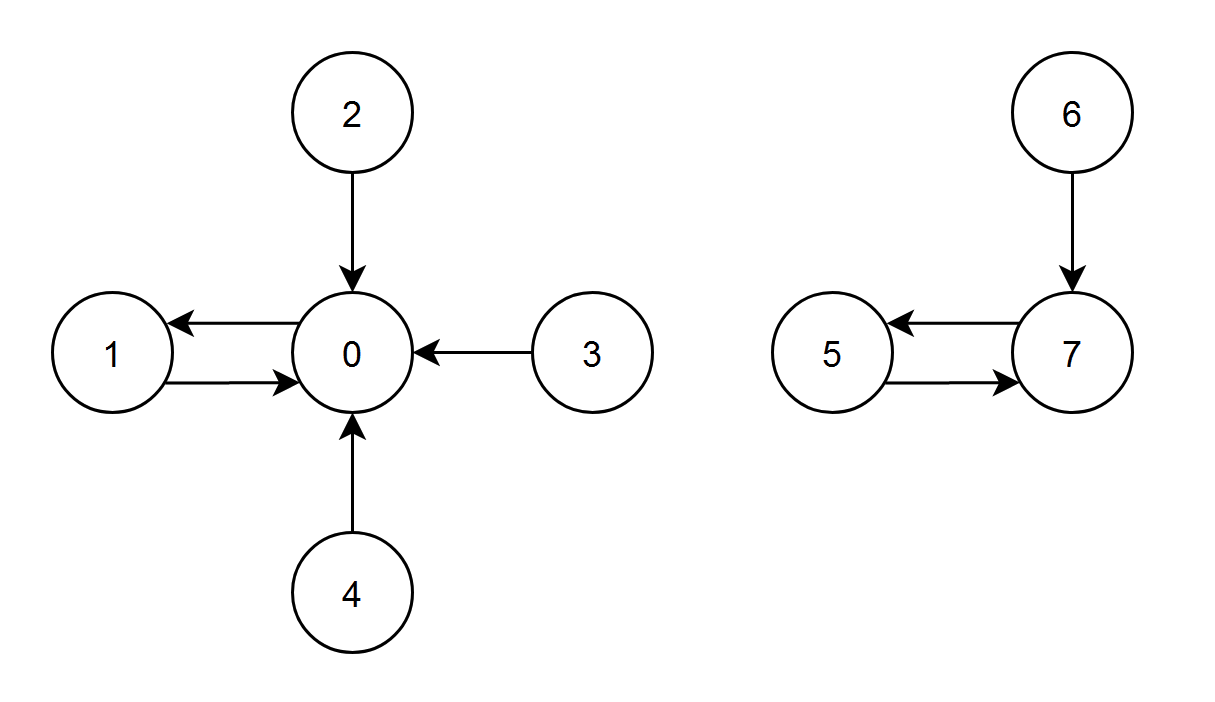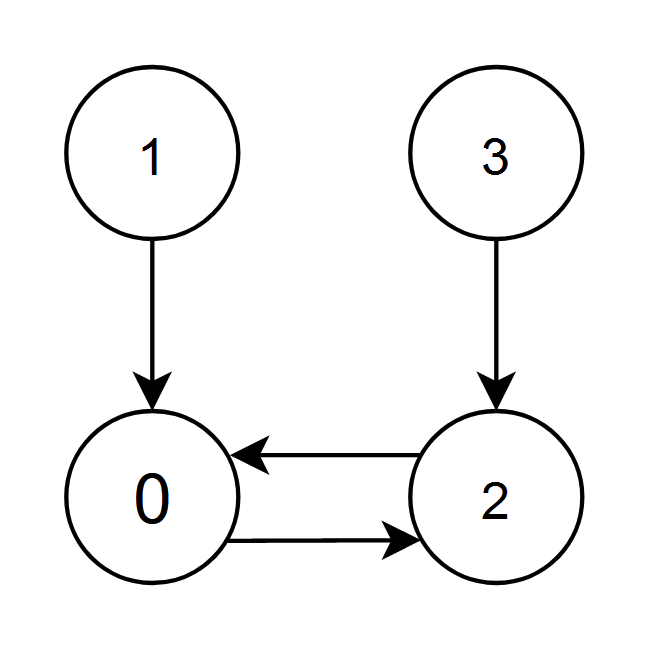2374. Node With Highest Edge Score
Medium
347
29

You are given a directed graph with `n` nodes labeled from `0` to `n - 1`, where each node has exactly one outgoing edge.

The graph is represented by a given 0-indexed integer array `edges` of length `n`, where `edges[i]` indicates that there is a directed edge from node `i` to node `edges[i]`.

The edge score of a node `i` is defined as the sum of the labels of all the nodes that have an edge pointing to `i`.

Return the node with the highest edge score. If multiple nodes have the same edge score, return the node with the smallest index.

Example 1:```Input: edges = [1,0,0,0,0,7,7,5]
Output: 7
Explanation:
- The nodes 1, 2, 3 and 4 have an edge pointing to node 0. The edge score of node 0 is 1 + 2 + 3 + 4 = 10.
- The node 0 has an edge pointing to node 1. The edge score of node 1 is 0.
- The node 7 has an edge pointing to node 5. The edge score of node 5 is 7.
- The nodes 5 and 6 have an edge pointing to node 7. The edge score of node 7 is 5 + 6 = 11.
Node 7 has the highest edge score so return 7.
```

Example 2:```Input: edges = [2,0,0,2]
Output: 0
Explanation:
- The nodes 1 and 2 have an edge pointing to node 0. The edge score of node 0 is 1 + 2 = 3.
- The nodes 0 and 3 have an edge pointing to node 2. The edge score of node 2 is 0 + 3 = 3.
Nodes 0 and 2 both have an edge score of 3. Since node 0 has a smaller index, we return 0.
```

Constraints:

• `n == edges.length`
• `2 <= n <= 105`
• `0 <= edges[i] < n`
• `edges[i] != i`
Accepted
26.6K
Submissions
57.1K
Acceptance Rate
46.6%

Seen this question in a real interview before?
1/4
Yes
No

Discussion (0)

Related Topics